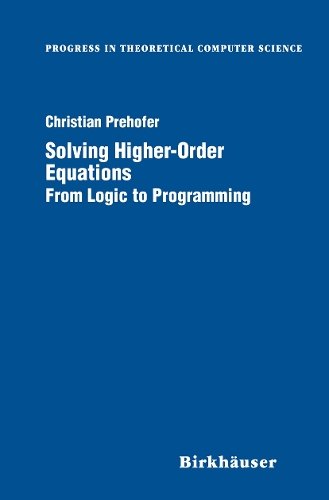•Solving Higher-Order Equations: From Logic to Programming - Progress in Theoretical Computer Science (Paperback)

(author)
£79.99
Paperback 188 Pages / Published: 21/10/2012
• We can order this

Usually dispatched within 3 weeks

This monograph develops techniques for equational reasoning in higher-order logic. Due to its expressiveness, higher-order logic is used for specification and verification of hardware, software, and mathematics. In these applica- tions, higher-order logic provides the necessary level of abstraction for con- cise and natural formulations. The main assets of higher-order logic are quan- tification over functions or predicates and its abstraction mechanism. These allow one to represent quantification in formulas and other variable-binding constructs. In this book, we focus on equational logic as a fundamental and natural concept in computer science and mathematics. We present calculi for equa- tional reasoning modulo higher-order equations presented as rewrite rules. This is followed by a systematic development from general equational rea- soning towards effective calculi for declarative programming in higher-order logic and A-calculus. This aims at integrating and generalizing declarative programming models such as functional and logic programming. In these two prominent declarative computation models we can view a program as a logical theory and a computation as a deduction.

Publisher: Springer-Verlag New York Inc.
ISBN: 9781461272786
Number of pages: 188
Weight: 320 g
Dimensions: 235 x 155 x 11 mm
Edition: Softcover reprint of the original 1st ed. 199